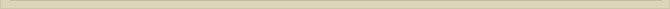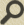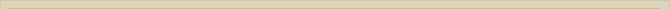## The Power of Σ in MS Excel

#### Excel is known to be a great analytical tool with many functions and formulas to manage your data efficiently. Excel is an extremely popular calculation tool and a very simple database inside an  advanced application. It offers easy to use environment which makes it a popular choice even for non-IT people who want to do all types of calculations on their data irrespective of its complexity. This is the reason why Excel is used in almost all offices for record-keeping, charting and graphing to take important decisions.

In this blog, I am going to talk about the formulas on simplest but interesting function of Excel i.e. Summation.

You might be using Excel for calculating wages, generating invoices, monitoring employees tasks, accounting and budgeting and for many such other purposes. Some simple formulas let you utilize the power of Excel at its best at your workplace. I am sure students, teachers and those who use Excel for all basic calculations will find this blog useful in their routine work.

Let me first take a minute to introduce Array for those who are not familiar with the terminology and its use. Array in Excel is a collection of values in a row, a column, or a combination of rows and columns. Array formulas enable you to perform complex calculations on a range of cells with standard worksheet functions. An array formula must be input in the following way:

• Select the range of cells where you want to enter your array formula
• Type in the array formula in first cell
• Press Ctrl + Shift + Enter

Excel automatically places curly braces { } around array formulas giving you the result in the range of cells you had selected.

Note: Do not attempt to type the curly braces yourself. Excel will not interpret the formula as an array formula.

You might have used Summation function Σ shown at the top right corner of toolbar in MS Excel for doing AutoSum i.e. one-click summation of your row or columnar data. When you click on the small drop-down besides Σ, it lets you perform 1-click calculations on other aggregate function like count, average, min and max.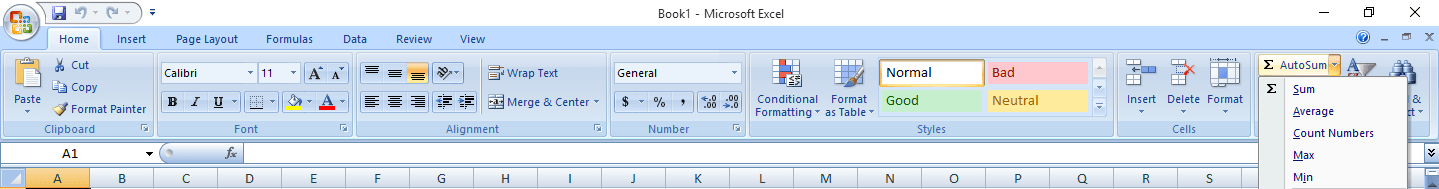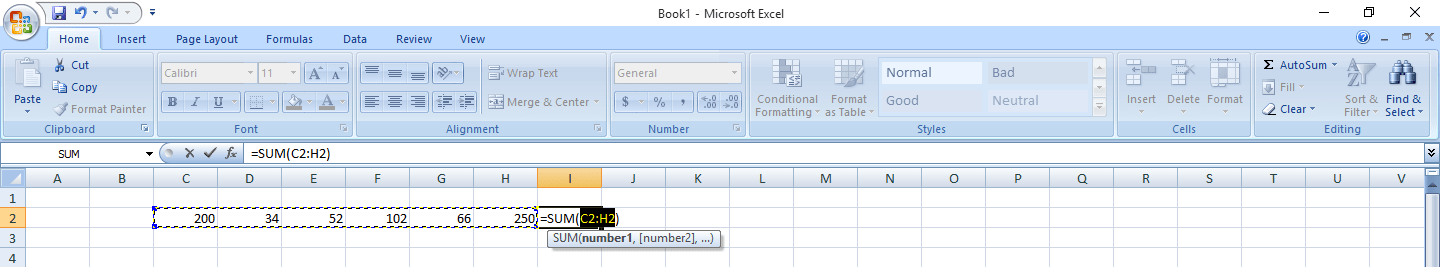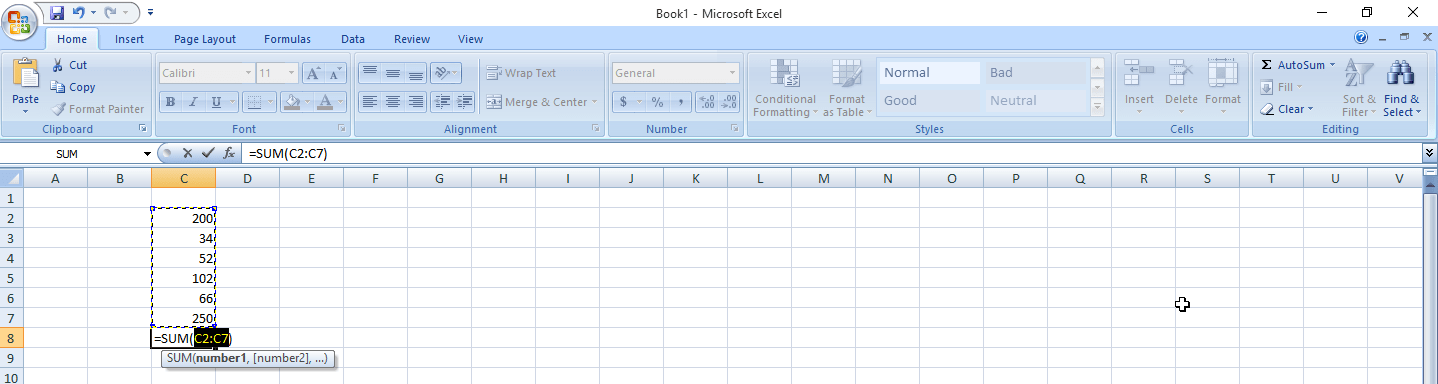If we look closely on how summation works, we will find that it is actually a mathematical function which sums up all the values in a given range.Which is essentiallyNow consider a scenario where you want to find sum of every alternate values in a row/column. If we look at the formula for summation, it will look like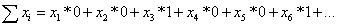To achieve this, we use the following formula in Excel

SUM(range*MOD(ROW(range),2)=0))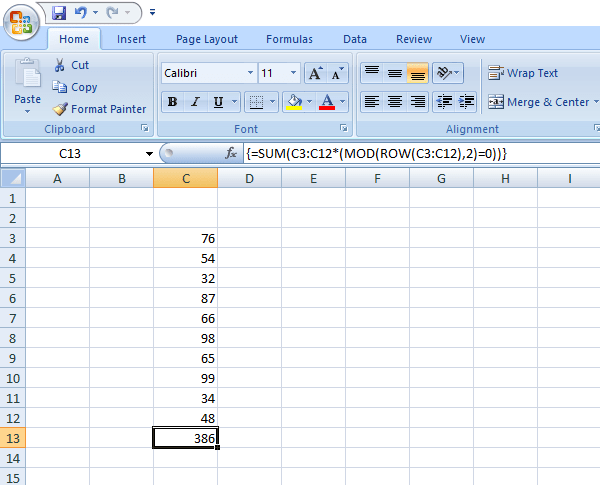Note: Notice the curly braces around the formula. This means that it’s an array formula and Ctrl + Shift + Enter has been pressed instead of Enter key after typing in the formula.

The same can be calculated using simple sum function, but it is not possible to select every alternate cell as an argument to sum function when you have hundreds of records.

Putting it in a simpler way for you to understand, MOD(ROW(range),2=0 checks the index of values in the given range returning TRUE (1) for all the even values and FALSE (0) for all the odd values. Now, multiplying your original array of values with this true/false array will result into alternate values whose sum yields our desired result.Moving ahead in the same fashion, you can also find sum of every 3rd value in a range applying the excel formula

ΣXi=SUM(range*MOD(ROW(range),3)=0))

to getHence, in generalized form, the excel array formula for finding the sum of every nth value in a range can be written as

SUM(range*MOD(ROW(range),n)=0))

Once you have understood the above technique, you can now also try an alternative to above formula in the form of

SUMPRODUCT((MOD(ROW(range),n)=0)*(range))

It is simple to use Microsoft Excel but understanding of such simple formulas can help you perform your calculations better and faster.

•By: Dr. Nidhi Arora

Nidhi Arora is a technophile and a fervent scholar with deep expertise in web technologies. She has been a dedicated enthusiast in exploring fundamentals of high-end technologies and has written many research papers in National and International journals.

•Visit the author’s website

•See all this author’s posts

### 4 Responses to “The Power of Σ in MS Excel”

1.Crystal says:

I am actually thankful to this web site for sharing this great article here.

2.Gosse says:

I like this post, enjoyed this one thanks for putting up.

3.Johnetta Mahfouz says:

he blog was how do i say it… relevant, finally something that helped me. Thanks

4.Rubenfeld says:

Whats Taking place i am new to this, I stumbled upon this I’ve found It positively useful and it has aided me out loads. I am hoping to give a contribution & assist different customers like its aided me. Good job.